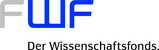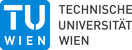# Discrete surfaces with prescribed mean curvature

Research project funded by the Austrian science fund FWF.

Project details

FWF project: P 29981

Livetime: 2017/09/01 - 2021/8/31

www:

People

 Project leader: Christian Müller Project members: Christian Müller Klara Mundilova

Abstract

Discrete surfaces have been investigated since thousands of years. The interest in those surfaces is theoretical, philosophical and also with a view towards applications. In particular in architecture and civil engineering appear discrete surfaces which can be seen as surfaces composed of individual facets. These facets can be planar triangles, quadrilaterals or other polygons. In some cases it makes even sense to consider non-planar facets.

A surface in three dimensional space, discrete or otherwise, has some curvature. There are several notions of curvature. In our project we are interested in the so called mean curvature. For example, a plane has mean curvature 0 whereas a sphere of radius r has mean curvature 1/r. A large radius implies a small curvature.

In the last decades discrete analogs of smooth curvature notions have been investigated. There are several discrete mean curvature notions which illustrates that the process of discretization is not always unique. The branch of mathematics that studies these objects and in which the present proposal is located is called “discrete differential geometry”.

It now seems natural to analyze a given discrete surface and to determine its curvature. Our primary objective is the reverse question. Suppose we are given any arbitrary mean curvature function. Is there a surface which has exactly that curvature? In smooth differential geometry this type of questions is by now very well studied even though there are still open questions. In the setting of discrete surfaces this question has been addressed only for two special cases which are geometrically important, though.

The question is, how does a surface, smooth or discrete, look like if the curvature is zero or any other constant everywhere. Both cases appear in nature. For example, as soap films in equilibrium. If the air pressure is equal on both sides of the surface then we have vanishing mean curvature and otherwise the mean curvature is any other constant number.

In our project we prescribe a mean curvature function which is not constant. A typical question can be the following. Suppose we are given a function which maps a value to every point in three dimensional space. This function will be our prescribed mean curvature function. Further we choose a curve in space which can be thought as a wire loop. Now investigate the existence of a surface which passes at the end through that curve such that in between the mean curvature value of that surface is exactly equal to the value of our prescribed function.

Publications:Canonical Möbius Subdivision
A. Vaxman, C. Müller and O. Weber
ACM Trans. Graphics (Proc. SIGGRAPH ASIA), 37(6), 2018.Folding the Vesica Piscis
K. Mundilova and T. Wills
In Eve Torrence et al., editors, Proceedings of Bridges 2018: Mathematics, Art, Music, Architecture, Education, Culture, (2018), 535-538.Discretizations of surfaces with constant ratio of principal curvatures
M.R. Jimenez, C. Müller and H. Pottmann
Discrete Comput. Geom.Regular Meshes from Polygonal Patterns
A. Vaxman, C. Müller and O. Weber
ACM Trans. Graphics (Proc. SIGGRAPH), 36(4), 2017.